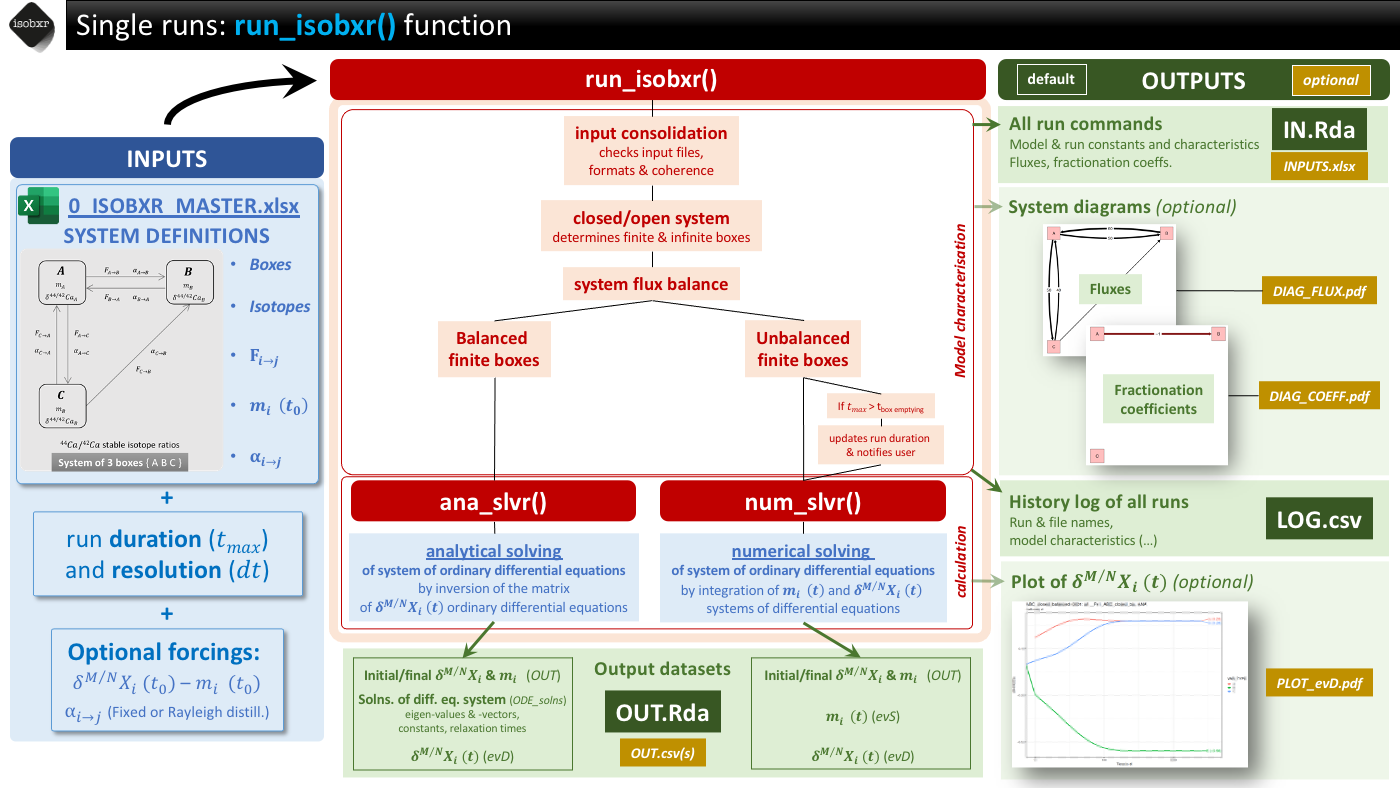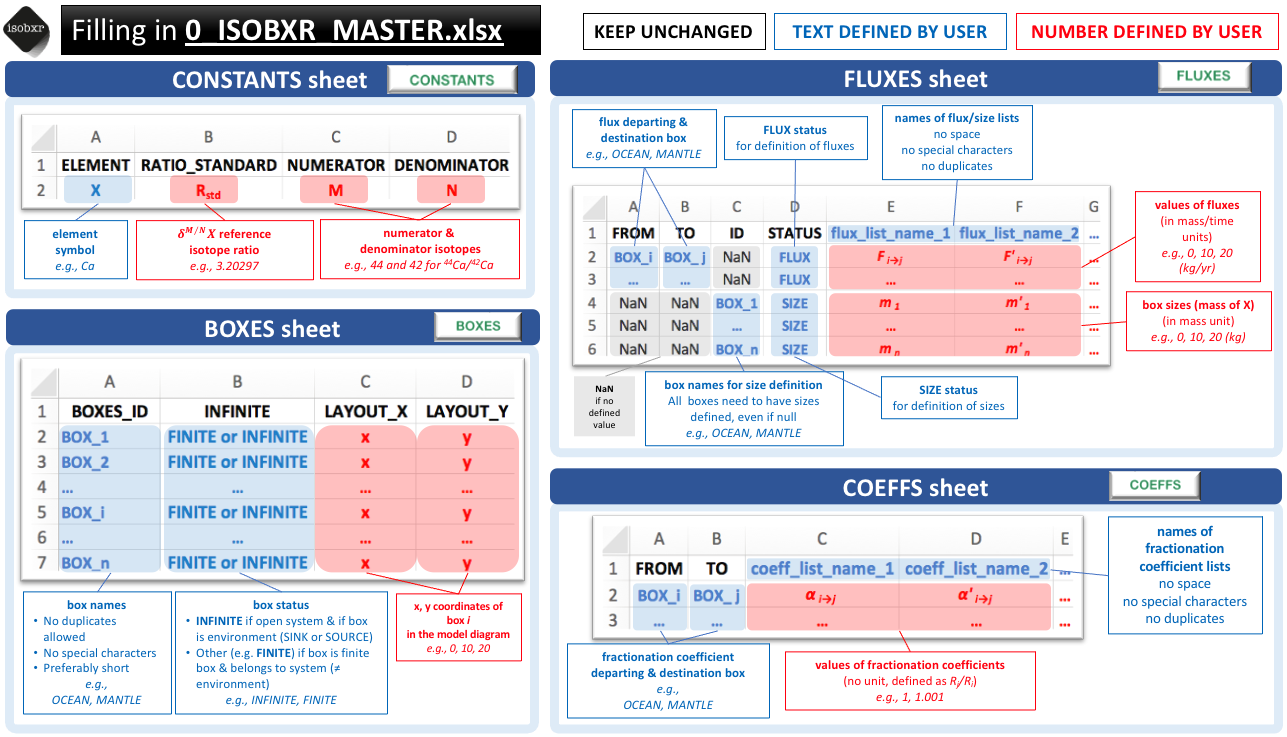# 3 - run_isobxr()

#### 2021-09-07

Full documentation and tutorials can be found on the isobxr website.

In this vignette, the user will be introduced to the main function of isobxr: run_isobxr.

Here, we shortly present the principles and general usage of the run_isobxr function.

The user is then invited to follow and run a step-by-step tutorial presenting several typical runs (4 - Run_isobxr: tutorial).

# System definitions

As a reminder, the systems considered in the isobxr package are described in the cheatsheet presented here and in the "General presentation" vignette.

# How does run_isobxr work?

The run_isobxr function is the main function of the isobxr package.

The run_isobxr function is used to perform single runs of stable isotope box models of all types of systems.

The run_isobxr function allows to:

1. assess the type of modelled system

2. render and archive the design of the model and run commands

3. automatically run num_slvr or ana_slvr solvers depending on the model design

4. provide user with the time evolutions of isotope compositions and masses of X in each box

The general workflow of the run_isobxr function is summarized in the cheatsheet below:To find the detailed description of run_isobxr inputs and outputs, the user can always refer to its documentation:

# To see documentation on run_isobxr function, type in console:
?run_isobxr

# Filling in 0_ISOBXR_MASTER.xlsx

The isobxr master file is the excel document containing all data defining the modelled system.

It provides the run_isobxr function with the main commands for a run of stable isotope box model.

Note
All the isobxr functions are meant to be run in relation with an isobxr master file. As run_isobxr is the fundamental function, called by the other isobxr functions, the isobxr master file is also required to run functions performing multiple runs (compose_isobxr, sweep_steady, sweep_dyn).

## Name and location

• The isobxr master file is an xlsx file
• The isobxr master file name needs to be exactly as follows: 0_ISOBXR_MASTER.xlsx
• The isobxr master file has to be stored in the working directory (workdir)

## Structure and format

The isobxr master file contains the 4 following sheet strictly named as follows:

1. CONSTANTS: Sheet defining the constants of the isotopic system
2. BOXES: Sheet defining names and status of all boxes constituting the system
3. FLUXES: Sheet defining the initial box sizes and mass fluxes of element X between boxes (structured as a column list)
4. COEFFS: Sheet defining the isotopic fractionation coefficients between all boxes (structured as a column list)

The general rules for the filling in of the isobxr master file are shown in cheatsheet below:More complete description of the master file filling can be found here and some examples and tutorials are shown here.

# Main arguments and outputs

The run_isobxr function takes required and optional arguments that are described in this section.

All arguments definitions of the run_isobxr function are accessible in its documentation called as follows

?run_isobxr

## Required arguments

The arguments required to run the run_isobxr function are exemplified and described here with the first tutorial example (see tutorial here):

run_isobxr(workdir = "/Users/username/Documents/1_ABC_tutorial",
SERIES_ID = "ABC_closed_balanced", # defining the name of the SERIES of run
flux_list_name = "Fx1_ABC_closed_bal", # calling the list of fluxes
coeff_list_name = "a1", #  calling the list of coefficients
t_lim = 2500, # running model for 1000 time units
nb_steps = 250, # running model with 100 steps
time_units = c("d", "yr")) # plot results in years as time units.
workdir
Working directory of 0_ISOBXR_MASTER.xlsx master file and where output files will be stored if saved by user (save_run_outputs = TRUE, default is FALSE).
SERIES_ID
Name of the model series the run belongs to. It determines the folder in which the output files will be stored if saved by user (save_run_outputs = TRUE, default is FALSE).
flux_list_name
Name of the list of fluxes and initial box sizes to be used for the run, calling (by its header name) a single column of the FLUXES sheet of the 0_ISOBXR_MASTER.xlsx file.
coeff_list_name
Name of the list of fractionation coefficients to be used for the run, calling (by its header name) a single column of the COEFFS sheet of the 0_ISOBXR_MASTER.xlsx file.
t_lim
Run duration, given in the same time units as the fluxes.
nb_steps
Number of calculation steps. It determines the resolution of the run.
time_units
• Vector defining the initial time unit (identical to unit used in fluxes), followed by the time unit used for the graphical output
• to be selected among the following: micros, ms, s, min, h, d, wk, mo, yr, kyr, Myr, Gyr
• e.g., c("d", "yr") to convert days into years

## Default and optional outputs

By default, the run_isobxr prints a summary plot of the run outputs (evolution of delta values and box sizes) in the R session.

All run_isobxr output data is stored in a temporary directory by default.

The user can save these outputs to their working directory by changing the save_run_outputs argument to TRUE.

The run_isobxr outputs are structured as shown in the full package documentation.

In addition to the default outputs, the run_isobxr function allows to:

• export system diagrams (as pdf, using to_DIGEST_DIAGRAMS = TRUE)
• export evolution of delta values (as pdf, using to_DIGEST_evD_PLOT = TRUE)
• export input and output data (as csv and xlsx, using to_DIGEST_CSV_XLS = TRUE)

These arguments are called as follows (using example used in tutorial):

run_isobxr(workdir = "/Users/username/Documents/1_ABC_tutorial",
SERIES_ID = "ABC_closed_balanced",
flux_list_name = "Fx1_ABC_closed_bal",
coeff_list_name = "a1",
t_lim = 2500,
nb_steps = 250,
time_units = c("d", "yr"),
to_DIGEST_DIAGRAMS = TRUE, # default is TRUE
to_DIGEST_evD_PLOT = TRUE, # default is TRUE
to_DIGEST_CSV_XLS = TRUE  # default is FALSE
)
Note
All run_isobxr outputs are not saved by default. Set save_run_outputs = TRUE to save outputs to a local working directory.

### Forcing initial box sizes

By default, run_isobxr sets the initial box sizes at the values found in the flux list.

It is possible to manually overwrite the initial size of one, several or all boxes for a given run performed by run_isobxr.

It is done by defining a data frame structured as follows.

FORCING_SIZE <-
data.frame(BOXES_ID = c("BOX_1", "...", "BOX_i", "..."),
SIZE_INIT = c("updated_size_1", "...", "updated_size_i", "..."))

FORCING_SIZE
#>   BOXES_ID      SIZE_INIT
#> 1    BOX_1 updated_size_1
#> 2      ...            ...
#> 3    BOX_i updated_size_i
#> 4      ...            ...

For the 3-boxes closed system model (ABC), in order to change the size of box C from 2000 mg of Ca (default as specified in isobxr master file for all flux lists of FLUXES sheet) to 3000 mg of Ca, the data frame should be structured as follows:

FORCING_SIZE <-
data.frame(BOXES_ID = c("C"),
SIZE_INIT = c(3000))

FORCING_SIZE
#>   BOXES_ID SIZE_INIT
#> 1        C      3000

### Forcing initial delta values

By default, the run_isobxr function sets the initial delta values of all boxes at 0 ‰.

It is possible to manually overwrite the initial delta values of one, several or all boxes for a given run performed by run_isobxr.
It is done by defining a data frame structured as follows.

FORCING_DELTA <-
data.frame(BOXES_ID = c("BOX_1", "...", "BOX_i", "..."),
DELTA_INIT = c("updated_delta_1", "...", "updated_delta_i", "..."))

FORCING_DELTA
#>   BOXES_ID      DELTA_INIT
#> 1    BOX_1 updated_delta_1
#> 2      ...             ...
#> 3    BOX_i updated_delta_i
#> 4      ...             ...

For the 3-boxes closed system model (ABC), in order to force initial isotope composition of box A to -1‰, and leave B and C initial values at 0‰, the data frame should be structured as follows:

FORCING_DELTA <-
data.frame(BOXES_ID = c("A"),
DELTA_INIT = c(-1))

FORCING_DELTA
#>   BOXES_ID DELTA_INIT
#> 1        A         -1

### Forcing fractionation coefficients

By default, the run_isobxr function sets the isotope fractionation coefficients at the values found in the coefficients list specified by user.

It is possible to manually overwrite the fractionation coefficients of one, several or all pairs of boxes for a given run performed by run_isobxr.
It is done by defining a data frame structured as follows.

FORCING_ALPHA <-
data.frame(FROM = c("BOX_i", "..."),
TO = c("BOX_j", "..."),
ALPHA = c("new_coeff_value", "..."),
FROM_TO = c("BOX_i_BOX_j", "..."))

FORCING_ALPHA
#>    FROM    TO           ALPHA     FROM_TO
#> 1 BOX_i BOX_j new_coeff_value BOX_i_BOX_j
#> 2   ...   ...             ...         ...

For the 3-boxes closed system model (ABC), in order to force the fractionation coefficient associated to the flux of Ca from box A to B to the value of 1.02, the data frame should be structured as follows:

FORCING_ALPHA <-
data.frame(FROM = c("A"),
TO = c("B"),
ALPHA = c(1.02),
FROM_TO = c("A_B"))

FORCING_ALPHA
#>   FROM TO ALPHA FROM_TO
#> 1    A  B  1.02     A_B

### Rayleigh distillation models

It is possible to overwrite isotope fractionation coefficients by defining their values as the result of Rayleigh type isotope distillation in the context of a fractional exchange at an interface.

We consider here the case of the loss of element X from a box A to a box C through an interface box B, all possibly part of a bigger box model system.

We suppose that the apparent fractionation coefficient $$\alpha_{A \to C}$$ associated to this flux $$F_{A \to C}$$ results from a Rayleigh type distillation occurring during the fractional exchange of element X at the interface box B.In this situation, the box A exchanges element X with box B (and possibly other boxes).

This is a fractional exchange, i.e. during this exchange, box A sends more of element X to box B than B sends back ($$F_{A \to B} > F_{B \to A}$$).

As we consider box B to be balanced, it loses the difference to box C: $$F_{B \to C} = F_{A \to B} - F_{B \to A}$$.

As a result, box A loses a total of $$F_{B \to C}$$ of element X per time unit.

We suppose here that the $$F_{A \to B}$$ that feeds box B is associated to no isotope fractionation.

On the other hand, we suppose that the $$F_{B \to A}$$ flux corresponding to the fractional loss of element X from interface box B, and returning to box A, is associated to an equilibrium or incremental isotope fractionation ($$\alpha^0 _{B \to A}$$).

The Rayleigh distillation model of isotopes thus predicts the following:

$R_{C} = R_{C, t_0} \dfrac{F_{B \to C}}{F_{A \to B}}^{\alpha^0 _{B \to A} - 1}$

The $$R_{C, t_0}$$ corresponds to the isotope ratio of the element X in box B before any exchange with box A occurs. It is thus equivalent to $$R_{A}$$ since the box B only input is via the $$F_{A \to B}$$ flux.

We thus can write the following definition of $$\alpha_{B \to C}$$ (equivalent here to $$\alpha_{A \to C}$$):

$$\alpha_{B \to C} = \dfrac{R_{C}}{R_{A}} = \dfrac{F_{B \to C}}{F_{A \to B}}^{\alpha^0 _{B \to A} - 1}$$

The run_isobxr function here takes as an optional input the data frame structured as follows:

FORCING_RAYLEIGH <-
data.frame(XFROM = c("B"), # Define the B>C flux at numerator
XTO = c("C"),
YFROM = c("A"), # Define the A>B flux at denominator
YTO = c("B"),
AFROM = c("B"), # Define the resulting fractionation coefficient
ATO = c("C"),
ALPHA_0 = c("a0") # Define the value of incremental B>A coefficient
)

FORCING_RAYLEIGH
#>   XFROM XTO YFROM YTO AFROM ATO ALPHA_0
#> 1     B   C     A   B     B   C      a0

The run_isobxr function will in this case overwrite the value of $$\alpha_{B \to C}$$ set in isobxr master file or using the FORCING_ALPHA parameter.

Indeed, if the user forced a new value for $$\alpha_{B \to C}$$ using the FORCING_ALPHA parameter, the run_isobxr function will prioritize the FORCING_RAYLEIGH parameter.

It is possible to define several Rayleigh distillation apparent fractionation coefficients in a given model.
This is done by adding rows to this data frame.